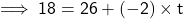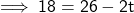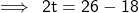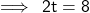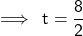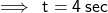## An automobile slows from 26 m/s to 18 m/s. Its acceleration is -2.0 m/s2. How much time does it take for the vehicle to slow dow

Question

An automobile slows from 26 m/s to 18 m/s. Its acceleration is
-2.0 m/s2. How much time does it take for the vehicle to
slow down?

in progress 0
6 months 2021-07-16T10:29:16+00:00 1 Answers 0 views 0

1. ### Given:–

Initial velocity,u = 26 m/s

Final velocity,v = 18 m/s

Acceleration,a = -2 m/s²

### Tobecalculated:-

Calculate the time take by a body.

### Solution:-

According to the first equation of motion: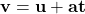★ Substituting the values in the above formula,we get: